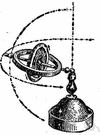# angular momentum

(redirected from Rotational angular momentum)
Also found in: Thesaurus, Medical, Encyclopedia.
Related to Rotational angular momentum: Rotational inertia, Rotational kinetic energy

## angular momentum

n.
1. The vector product of the position vector (from a reference point) and the linear momentum of a particle.
2. The vector sum of the angular momentums of each infinitesimal component particle of an extended body.

## angular momentum

n
(Mathematics) a property of a mass or system of masses turning about some fixed point; it is conserved in the absence of the action of external forces

## an′gular momen′tum

n.
the product of the moment of inertia of a body about an axis and its angular velocity with respect to the same axis.
[1870–75]

## an·gu·lar momentum

(ăng′gyə-lər)
A quantity used to measure the motion of a body that is moving in a circle. The angular momentum depends on the mass and velocity of the body, and on the radius of the circle that it is moving along. Because the velocity of a body moving in a circle is always changing (since velocity is speed and direction), its angular momentum is also always changing.
ThesaurusAntonymsRelated WordsSynonymsLegend:
 Noun 1angular momentum - the product of the momentum of a rotating body and its distance from the axis of rotation; "any rotating body has an angular momentum about its center of mass"; "angular momentum makes the world go round"momentum - the product of a body's mass and its velocity; "the momentum of the particles was deduced from meteoritic velocities"
Translations
moment hybnosti
kotni moment
ugaoni moment
References in periodicals archive ?
During evolution first planets were formed when the orbital angular momentum of the planet is compared to the rotational angular momentum of the central body.
A tidal antipode is an equilibrium-restoring mechanism to maintain Earth's rotational angular momentum, where both the sun and the moon are the disrupting bodies dynamically and visibly affecting Earth's oceans.

Site: Follow: Share:
Open / Close